# Publications

• #### « Stable GSTC formulation for Maxwell's equations »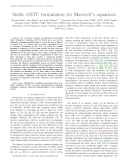Status: submitted (2021) [ preprint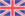]

Authors: Nicolas Lebbe, Kim Pham and Agnès Maurel

Keywords: Metasurface · GSTC · Two-scale homogenization · Finite element simulation

Abstract:... We revisit the classical zero-thickness Generalized Sheet Transition Conditions (GSTCs) which are a key tool for efficiently designing metafilms able to control the flow of light in a desired way. It is shown that it is more convenient to use an enlarged formulation of the GSTC in which the original metafilm is replaced by GSTCs that exclude the layer from the physical or computational domain. These new "layer" transition conditions have the same form as their "sheet" analogues hence they do not necessitate additional complications in their use; their advantage is that they provide a well-posed problem hence guaranty the stability of numerical schemes in the timedomain. These assessments are demonstrated for an all-dielectric structure; the effective susceptibility tensors are derived thanks to asymptotic analysis combined with homogenization technique and bounds for the susceptibilities entering the balance of energy are provided. While negative constant susceptibilities appear in the classical zero-thickness GSTCs, their values in the enlarged formulation are always positive which ensure the stability of the effective problem. Validation of the effective model is provided by means of comparison with direct numerics in two and three dimensions.

• #### « High-efficiency and broadband photonic polarization rotator based on multilevel shape optimization »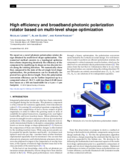Status: published in Optics Letters, Volume 44, no. 8, pp. 1960–1963 (2019) [ article, preprint]

Authors: Nicolas Lebbe, Alain Glière and Karim Hassan

Keywords: Shape optimization · Photonic · Polarization rotator · Multi-levels

Abstract:... We report on a novel photonic polarization rotator design obtained by multi-level shape optimization. The numerical method consists in a topological optimization scheme improving iteratively the efficiency of the component by modifying its shape on two discrete levels along the etching direction. We numerically show that, compared to the state of the art single level shape optimization, the performances can be drastically improved for a given device length. Next, the polarization conversion efficiency can be further improved up to a computed value of ~98.5 % with less than 0.35 dB insertion losses on a 100 nm bandwidth for a 6 μm × 1 μm footprint.

• #### « Optimization of the shape of regions supporting boundary conditions »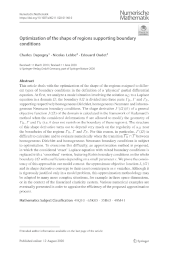Status: published in Numerische Mathematik, Volume 146, pp. 51–104 (2020) [ article, preprint]

Authors: Charles Dapogny, Nicolas Lebbe and Edouard Oudet

Keywords: Shape optimization · PDE · Boundary conditions · Level-set method

Abstract:... This article deals with the optimization of the shape of the regions assigned to different types of boundary conditions in the definition of a 'physical' partial differential equation. At first, we analyze a model situation involving the solution $$u_\Omega$$ to a Laplace equation in a domain $$\Omega$$; the boundary $$\partial \Omega$$ is divided into three parts $$\Gamma_D$$, $$\Gamma$$ and $$\Gamma_N$$, supporting respectively homogeneous Dirichlet, homogeneous Neumann and inhomogeneous Neumann boundary conditions. The shape derivative $$J^\prime(\Omega)(\theta)$$ of a general objective function $$J(\Omega)$$ of the domain is calculated in the framework of Hadamard's method when the considered deformations $$\theta$$ are allowed to modify the geometry of $$\Gamma_D$$, $$\Gamma$$ and $$\Gamma_N$$ (i.e. $$\theta$$ does not vanish on the boundary of these regions). The structure of this shape derivative turns out to depend very much on the regularity of $$u_\Omega$$ near the boundaries of the regions $$\Gamma_D$$, $$\Gamma$$ and $$\Gamma_N$$. For this reason, in particular, $$J^\prime(\Omega)$$ is difficult to calculate and to evaluate numerically when the transition $$\overline{\Gamma_D} \cap \overline{\Gamma}$$ between homogeneous Dirichlet and homogeneous Neumann boundary conditions is subject to optimization. To overcome this difficulty, an approximation method is proposed, in which the considered 'exact' Laplace equation with mixed boundary conditions is replaced with a 'smoothed' version, featuring Robin boundary conditions on the whole boundary $$\partial \Omega$$ with coefficients depending on a small parameter $$\varepsilon$$. We prove the consistency of this approach in our model context: the approximate objective function $$J_\varepsilon(\Omega)$$ and its shape derivative converge to their exact counterparts as $$\varepsilon$$ vanishes. Although it is rigorously justified only in a model problem, this approximation methodology may be adapted to many more complex situations, for example in three space dimensions, or in the context of the linearized elasticity system. Various numerical examples are eventually presented in order to appraise the efficiency of the proposed approximation process.

• #### « Shape optimization for the design of passive mid-IR photonic components »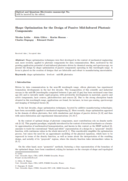Status: published in Optical and Quantum Electronics, Volume 51, no. 5, pp. 166–179 (2019) [ article, preprint]

Authors: Nicolas Lebbe, Alain Glière, Karim Hassan, Charles Dapogny and Edouard Oudet

Keywords: Shape optimization · Level-set · Mid-IR photonics

Abstract:... Shape optimization techniques were first developed in the context of mechanical engineering and, more recently, applied to photonic components for data communication. Here, motivated by the growing application potential of mid-infrared photonics driven by chemical sensing and spectroscopy, we present the design by shape optimization of passive components operating in this wavelength range. A focus is placed on the creation of designs that are fabricable and robust to manufacturing uncertainties.

• #### « Robust shape and topology optimization of nanophotonic devices using the level set method »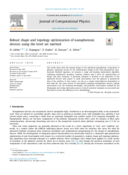Status: published in Journal of Computational Physics, Volume 395, pp. 710–746 (2019) [ article, preprint]

Authors: Nicolas Lebbe, Charles Dapogny, Edouard Oudet, Karim Hassan and Alain Glière

Keywords: Shape optimization · Level-set method · Nanophotonics · Robustness · Maxwell equations

Abstract:... This paper deals with shape optimization of nanophotonic devices subject to some environmental or fabrication uncertainties. In order to obtain robust components according to such uncertainties, we devised a method based on a multi-objectives algorithm together with the classical level-set shape optimization. A particular attention was paid to the case of manufacturing variations coming from the etching and lithography steps that the integrated optics community considers to be of major importance in the design of nanophotonic devices. Our article presents numerous numerical examples validating on the one hand our multi-objectives algorithm and on the other hand the robust optimization procedure.

• #### « Regularized decomposition of large scale block-structured robust optimization problems »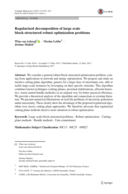Status: published in Computational Management Science, Volume 14, no. 3, pp. 393–421 (2017) [ article, preprint]

Authors: Wim van Ackooij, Nicolas Lebbe and Jérôme Malick

Keywords: Large scale block-structured problems · Robust optimization · Cutting-plane methods · Bundle methods · Unit-commitment

Abstract:... We consider a general robust block-structured optimization problem, coming from applications in network and energy optimization. We propose and study an iterative cutting-plane algorithm, generic for a large class of uncertainty sets, able to tackle large-scale instances by leveraging on their speciﬁc structure. This algorithm combines known techniques (cutting-planes, proximal stabilizations, efﬁcient heuris-tics, warm-started bundle methods) in an original way for better practical efﬁciency. We provide a theoretical analysis of the algorithm and connections to existing literature. We present numerical illustrations on real-life problems of electricity generation under uncertainty. These clearly show the advantage of the proposed regularized algorithm over classic cutting plane approaches. We therefore advocate that regularized cutting plane methods deserve more attention in robust optimization.